Next: dunai2001sp.m Up: Subsidiary calculation functions Previous: d2r.m   Contents

## desilets2006sp.m

Syntax:

scalingfactor = desilets2006sp(h,Rc)


Calculates the geographic scaling factor for cosmogenic-nuclide production for particular cutoff rigidity and atmospheric pressure according to the scheme in:

Desilets D., Zreda M., Prabu T., 2006. Extended scaling factors for in situ cosmogenic nuclides: New measurements at low latitude. Earth and Planetary Science Letters, v. 246, pp. 265-276.

The input arguments are h, atmospheric pressure (hPa), and Rc, cutoff rigidity (GV). Accepts vector arguments.

This function:

Converts atmospheric pressure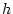(hPa) to atmospheric depth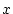(g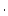cm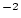) by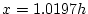.

Assigns cutoff rigidities below 2 GV a value of 2 GV.

Obtains the effective attenuation length in air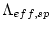via Equation (4) in the source paper: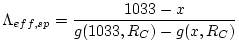(26)

where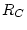is the cutoff rigidity (GV) and the function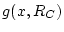is: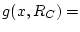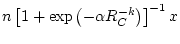(27)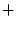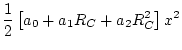(28)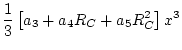(29)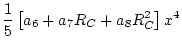(30)

given constants: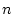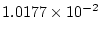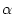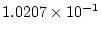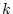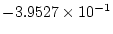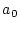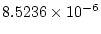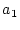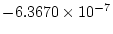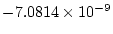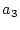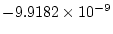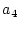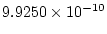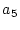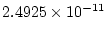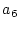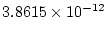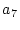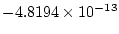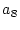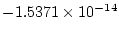Obtains the altitude scaling factor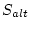: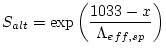(31)

Obtains the latitude scaling factor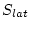via Equation (6) in the source paper: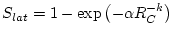(32)

whereis the cutoff rigidity,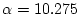, and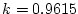.

Finally, obtains the total scaling factor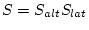.

Accepts either scalars or vectors of equal sizes for all the input arguments. Returns either a scalar or a vector of the appropriate size.Next: dunai2001sp.m Up: Subsidiary calculation functions Previous: d2r.m   Contents
2007-11-13